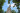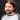# The Caesar's cipher

February 10th, 2020How did it get so late so soon? - Dr. Seuss

Let’s jump-start the blog again and explore one of the first exercises I worked on when learning Elixir: a simple substitution cipher, in this case the Caesar cipher and what’s the basic math required to create it.

## What is a substitution cipher?

A substitution cipher, in simple terms, is a method of encrypting text by substituting the letters for another letters. One of the simplest ciphers is the Caesar cipher, in which we shift each letter by some fixed number. For example, if we replace a letter with the next letter after it, `zebra` would become `afcsb`. Replacing each letter of `rosebud` with the 13th letter after it, we’d obtained `ebfrohq`, this last example is called ROT-13 (rotate by 13 places).

You might have noticed that z becomes a in zebra, and r becomes e in the word rosebud. When we go beyond the last letter, we simply go to the start, the alphabet wraps around when reaching the end.

## Building the cipher

The english alphabet has 26 letters, by assigning a number to each of them, we have the range [0, 25]: `a=0, b=1, ..., z=25`

Let’s try substituting y by the 13th letter after it:

``````y + 13 = l
24 + 13 = l
l = 37``````

We already know that `l = 11`, not 37, there’s something wrong here. What we need is a way to “wrap around” and start from the beginning. Luckily, we use something that works that way in our daily lives: regular time and military time.

### What time is it?

What do we mean when we say 17:00? If we go to regular time, we mean 5:00, what about 24:00? 00:00. When converting military time to regular time, we can imagine the former wrapping around after 12:00. The same thing happens in regular time, if we add two hours to 11:00, we don’t get 13:00, but 01:00.In mathematics, this concept is called modular arithmetic, in which numbers wrap around after reaching a certain value, the modulus. In regular time, the modulus is 12. Using the modulo operation:

``````// JavaScript
console.log(23 % 12)  // 11``````
`````` // Elixir
IO.puts rem(17, 12) # 5
``````

Coming back to the alphabet, the modulus is 26:

``````// l = 11

y = 24
IO.puts rem(y + 13, 26) # 11``````

### Implementing a one letter cipher

Now that we know how to wrap around and get the right letter, there’s something more to consider: character codepoints.

``````// Javascript
console.log("a".codePointAt(0)); // 97``````
``````// Elixir
IO.puts ?a # 97``````

The character a is 97, not 0, and while the cipher will work if we don’t go beyond z, we need to implement the wrap around functionality:

``````// Javascript
const z = "y".codePointAt(0) + 1 // 122
console.log(String.fromCodePoint(z)) // "z"

console.log(String.fromCodePoint(z + 1)) // "{"``````
``````// Elixir
z = ?y + 1 # 122
IO.puts [z] # 'z'

IO.puts [z + 1] # '{'``````

Let’s implement it and see what happens:

``````// Javascript
const z = "z".codePointAt(0)
const a = "a".codePointAt(0) // 97

const hopefullyA = (z + 1) % 26 // 19``````
``````// Elixir
hopefully_A = ?z + 1 |> rem(26) # 19 ``````

We’re getting somewhere, but a is not 19, why? The range is different, in Unicode codepoints `a = 97, b = 98, ..., z = 122`, in our implementation we’ve been using the range [97, 122], not [0, 25], to make this work we have to normalise the former range:

``````// Javascript
const a = "a".codePointAt(0) // 97

const z = "z".codePointAt(0) - a

console.log((z + 1) % 26) // 0``````
``````// Elixir
IO.puts ?z + 1 - ?a |> rem(26) # 0``````

Wonderful! We now get the correct range, in which every number gives us the distance from a. With this knowledge, we can get the codepoint of the shifted letter:

``````// Javascript
const codePointOfA = "a".codePointAt(0)
const normalisedZ = "z".codePointAt(0) - codePointOfA

const shiftedZ = codePointOfA + (normalisedZ + 1) % 26

console.log(String.fromCodePoint(shiftedZ)) // "a"``````
``````// Elixir
shiftedZ = ?a + rem(?z + 1 - ?a, 26)

IO.puts [shiftedZ] # 'a'``````

## Summary

Hopefully this simple exploration of the substitution cipher has been entertaining. For me it’s been fun to work on this exercise and the post, trying to make my rationale as atomic as possible pieces and, specially, figuring out how to deal with strings and codepoints in Elixir, which might deserve another article in the future.

Of course, this is not the end of the exercise, we’d still need to cater for more than one letter and implement the cipher for lower and upper case letters, among other tiny things. If we want to simplify it even more and assuming the number used to substitute is fixed, as in ROT-13, we could use a dictionary, avoiding any calculation, but then the exercise wouldn’t have been as fun.Written by Alejandro Napoles. Software engineer.